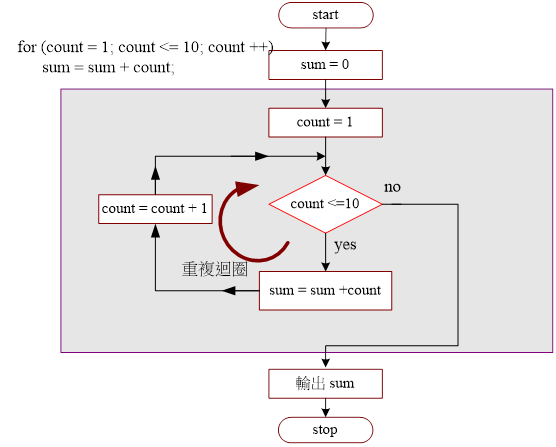Java 程式設計(一)  ：第 五章 重複性流程• 5-1 重複性流程簡介

• 5-2 for 迴圈控制

• 5-2-1 for 迴圈敘述

• 5-2-2 範例研討：連續累加程式

• 5-2-3 自我挑戰：累加程式變化

• 5-2-4 範例研討：連續累乘程式

• 5-2-5 自我挑戰：九九乘法評分系統

(1)  for 迴圈（for loop

(2) while 迴圈（while loop

(3) do/while 迴圈（do/while loop

5-2 for 迴圈控制

5-2-1 for 迴圈敘述5-1 for 敘述的流程圖

 語法： 範例：由 1 累加到 10 for (條件初始; 條件判斷; 增減量 ) { Statement 1; Statement 2, ….. } int sum = 0; for (k=1; k <= 10; k = k +1) { sum = sum + k;   //for body } System.out.println("Sum = " + sum);

For 敘述的小括號內包含 3 個敘述句，之間以『分號』（;）分隔。第一個敘述句為條件變數的初值設定（如 k = 1）；第二個為測試變數內容是否滿足條件（如 k < =10）；第三個敘述句為執行 for 區塊敘述（如 sum = sum + k）後，再針對條件變數做增量或減量的變動（如 k = k +1）。另一重點，如果區塊敘述超過一行的話，則必須利用左右大掛號包起來（{ ….}）。

A）程式功能：

 1 + 2 + .. + 10 = 55

B）製作技巧研討：5-2 累積器功能5-3 連續累加流程圖

 次數 count count <=10 ? sum = sum +count count = count + 1 1 1 yes sum = 0 +1 =1 count = 1+1=2 2 2 yes sum = 1 +2 =3 count = 2+1=3 3 3 yes sum = 3 +3 =6 count = 3+1=4 4 4 yes sum = 6 +4 =10 count = 4+1=5 5 5 yes sum = 10 +5 =15 count = 5+1=6 6 6 yes sum = 15 +6 =21 count = 6+1=7 7 7 yes sum = 21 +7 =28 count = 7+1=8 8 8 yes sum = 28 +8 =36 count = 8+1=9 9 9 yes sum = 36 +9 =45 count = 9+1=10 10 10 yes sum = 45 +10 =55 count = 10+1=11 11 11 no

C）程式範例：Ex5_1.java

 01 02 03 04 05 06 07 08 09 10 11 12 // Ex5_1.java   public class Ex5_1 {      public static void main(String args[]) {           int count, sum;           sum = 0;           for (count = 1;count <= 10; count = count +1) {                 sum = sum + count;           }           System.out.printf("1 + 2 + .. + 10 = %d\n", sum);      } }

D）程式重點分析：

• 6 sum = 0;。累加器初值設定為 0

• 7~9 for (….) { …..}For 敘述句，其中 count = count + 1 可改為 count++；又敘述區塊僅有一條敘述句，則左右大括號（{ … }）可以省略。

• 8 sum = sum + count;。累加計算功能，將原來 sum 變數的內取出與 k 相加後，再存入 sum 變數內。

(A)程式變化()PM5_1_1.java

 1  + 2 + .. + 1000 = 500500

(B)程式變化()PM5_1_2.java

sum = 100 + 99 …, + 1，執行結果如下：

 100  + 99 + .. + 1 = 5050

(C)程式變化()PM5_1_3.java

 0   + 2 + .. + 1000 = 250500

(D)程式變化()PM5_1_4.java

 1 + 3 + .. + 999 = 250000

A）程式功能：Ex5_2.java

 total * 1 = 1 total * 2 = 2 total * 3 = 6 total * 4 = 24 total * 5 = 120 total * 6 = 720 total * 7 = 5040 total * 8 = 40320 total * 9 = 362880 total * 10 = 3628800

B）製作技巧研討：

 宣告計數器（count，1, 2, …, 10）； 宣告累積器並設定初值為 1（total = 1）； for (count = 1; count <= 10; count++) { total = total * count; 列印 total, count; }

C）程式範例：

 01 02 03 04 05 06 07 08 09 10 11 12 // Ex5_2.java   public class Ex5_2 {    public static void main(String args[]) {        int count, total;        total= 1;        for (count = 1;count <= 10; count++) {             total = total * count;             System.out.printf("total * %d = %d\n", count, total);        }    } }

D）程式重點分析：

• 7~10 for(count = 1; count<=10; count++) { ..}For 敘述句，條件初始值設定為 1，當它小於或等於 10 則執行 for 程式區塊，執行完後再執行 count++（增量 1），再回去測試條件是否成立；如條件不成立，則離開 for 迴圈敘述。

• 8 total = total * count;。累乘程式功能，首先將 total 內容取出，與 count 相乘後結果，再存回 total 變數內。

A）程式功能：PM5_2.java

 == 歡迎進入九九乘法評分系統 == 請輸入 9 * 7 = 63 請輸入 3 * 7 = 21 請輸入 3 * 3 = 9 請輸入 8 * 3 = 24 請輸入 6 * 3 = 15 錯了!! 答案是 18 請輸入 5 * 9 = 45 請輸入 3 * 2 = 7 錯了!! 答案是 6 請輸入 8 * 6 = 48 請輸入 3 * 7 = 21 請輸入 1 * 5 = 5 您得到 80 分

B）製作技巧提示：

 導入相關套件（import java.util.*;）; 宣告與產生亂數物件（Random random） 宣告與產生輸入物件（Scanner keyin）; 宣告相關變數； for (count = 1; count <= 10; count++) { 產生第一個 1 ~ 9 亂數（ran1 = 1 + random.nextInt(9)）; 產生第二個亂數（ran2）; 計算正確答案（value = ran1 * value2） 顯示兩亂數並要求輸入答案（ran1, ran2）; 讀入學生答案（answer = keyin.nextInt()）; if (answer == value) 累加學生答對題數（correct = correct + 1）; else 顯示學生答錯了, 並給予正確答案; } 列印學分得到分數（correct * 10）;

Java 程式設計(一) 含程式邏輯